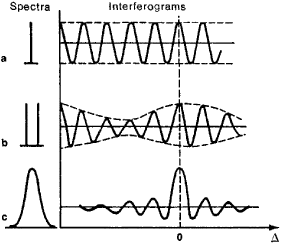# Fourier-Transform Spectroscopy

## Fourier-Transform Spectroscopy

a method of optical spectroscopy in which spectra are obtained by two procedures. First, an interferogram of the radiation in question is taken; then, the spectrum of the radiation is computed by means of the Fourier transform of the interferogram.

In Fourier-transform spectroscopy, interferograms are obtained by means of a Michelson interferometer, which is adjusted to produce interference fringes of equal inclination in the plane of the exit pupil (see Figure 1 in INTERFEROMETER). When one of the interferometer mirrors is shifted linearly, the optical path difference Δ in the arms of the interferometer is altered. As Δ varies, the radiation in question is modulated; the modulation frequency f depends on the rate v of variation of Δ and on the wavelength γ or the wave number v = l/λ of the radiation. Maxima of the radiation intensity occur for Δ = kλ (k = 0, 1, 2, . . .), and intensity minima occur for Δ = kλ/2. If v = const, f = v/λ = vv; that is, each wavelength of the radiation in question is coded by a specific f.

The signal at the detector—that is, the interferogram—is a set of sinusoidal wave trains (see Figure 1). A specific interferogram corresponds to each individual spectrum. In some cases, the spectrum may be determined directly from the interferogram. However, in most cases, a harmonic analysis of the interferogram must be performed to transform it into a spectrum. For this purpose, the interferogram is represented as a series of digits that correspond to discrete values of the radiation intensity as the path difference is varied from 0 to Δmax or from – Δmax to + Δmax over equal intervals. The series, which contains between 102 and 106 values in various instruments, is entered into the memory of a computer. The computer employs a Fourier transform to calculate the spectrum in a time interval ranging from several seconds to several hours, depending on the complexity of the spectrum and the number of values in the series.Figure 1. Interferograms corresponding to: (a) a spectral line, (b) a spectral doublet, (c) a spectral band

The set of equipment that produces and transforms interferograms is called a Fourier spectrometer. As a rule, such a spectrometer consists of a two-beam interferometer, a condenser, a radiation detector, a system for measuring Δ, an amplifier, an analog-to-digital converter, and a computer that is either built into the instrument or located at a computer center. The complexity with which spectra are obtained by means of a Fourier spectrometer is compensated by the advantages of the spectrometer with respect to other spectroscopic instruments. For example, a Fourier spectrometer may be used for the simultaneous recording of an entire spectrum. Fourier spectrometers have a higher light power than spectroscopic instruments containing a dispersing element and having the same resolution, because an entrance pupil that is larger than the slit of such an instrument can be used in an interferometer. The higher light power makes it possible to reduce the time required for recording spectra, to improve the signal-to-noise ratio, to increase the resolution, and to reduce the size of the spectrometer. The computer in the spectrometer may be used not only to calculate the spectrum but also to perform other operations with respect to the processing of the experimental data and to control and monitor the operation of the spectrometer.

Fourier-transform spectroscopy is used mostly in research where other methods are ineffective or altogether inapplicable, mainly in the infrared region of the spectrum. For example, the near-infrared spectra of certain planets have been recorded in a few hours, whereas several months would have been required to record the same spectra by means of a spectroscopic instrument with a dispersing element. Miniaturized Fourier spectrometers have been used in studies from space of the earth’s surface and near-terrestrial space in the intermediate-infrared region. Laboratory Fourier spectrometers for the far-infrared region are employed in chemistry. Fourier spectrophotometers (seeSPECTROPHOTOMETER) for the entire infrared region have also been constructed.

### REFERENCES

Bell, R. J. Vvedenie v fur’e-spektroskopiiu. Moscow, 1975. (Translated from English.)
Infrakrasnaia spektroskopiia vysokogo razresheniia: Sb. Moscow, 1972. (Translated from French and English.)
Mertz, L. Integral’nye preobrazovaniia v optike. Moscow, 1969. (Translated from English.)

B. A. KISELEV

Site: Follow: Share:
Open / Close